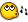## Recommended Posts

Hi

i have a array list like " 2 7 8 9 14 20 24 25 29 35 "

i must create a gui window with radio butons with elements form array ( 2, 7, 8 etc)

the minimum number of radio buttons is 1 and the max is 32

can you help me with that?

##### Share on other sites

Hi

i have a array list like " 2 7 8 9 14 20 24 25 29 35 "

i must create a gui window with radio butons with elements form array ( 2, 7, 8 etc)

the minimum number of radio buttons is 1 and the max is 32

can you help me with that?

Something like this?

#Include <GUIConstants.au3>

Dim $array = [2, 7, 8, 9, 14, 20, 24, 25, 29, 35] GUICreate('Radio', 200, UBound($array)*19)

For $i = 0 To UBound($array)-1
GUICtrlCreateRadio($array[$i], 10, 10+($i*17)) Next GUISetState() Do$msg = GUIGetMsg()
Until $msg =$GUI_EVENT_CLOSE
##### Share on other sites

great

is exactry what i need

but i still have some questions ( i am not so good in autoit)

i have this file c:\check.dat (you have the uploaded file , you must change the extension from.txt to .dat)

the values from that file are generated ( numbers from 1 to 32)

i make a array

CODE
Dim $aRecords$list= _FileReadToArray("c:\check.dat", $aRecords) FileWrite ("c:\test1.txt",$lista) ; to check if the array is ok

after that

CODE
$number= _FileCountLines ("c:\check.dat") to see the number of lines fro file after CODE For$x = 1 to $aRecords$right=StringTrimleft ($aRecords[$x], 4)

FileWrite ("c:\right",$right) ; to verify if the trim was good i trim each line to extract the value of " Key: " after that CODE Dim$array[$number] = [$right]

GUICreate('Radio', 200, UBound($array)*19) that is fro your code, i customized a little , to give the number of radio buttons form gui i stuck hereCODE For$i = 0 To UBound($array)-1 GUICtrlCreateRadio($array[$i], 10, 10+($i*17))

i dont know how to make the radio buttons with the values from $right that i explained few line up check.txt Edited by erikson ##### Link to comment ##### Share on other sites Nobody? ##### Link to comment ##### Share on other sites • 1 month later... Sorry for the delayed response ... I've been away from the forum for a bit, and you may have figured this out already. How about this, I put some comments in your code further down (with the @ @)#include <GUIConstants.au3> #include <File.au3> Dim$array
_FileReadToArray('check.dat', $array) GUICreate('Radio', 200, (UBound($array)-1)*19)

For $i = 1 To UBound($array)-1
$array[$i] = StringTrimLeft($array[$i], 5)
GUICtrlCreateRadio($array[$i], 10, 10+(($i-1)*17)) Next GUISetState() Do$msg = GUIGetMsg()
Until $msg =$GUI_EVENT_CLOSE

great

is exactry what i need

but i still have some questions ( i am not so good in autoit)

i have this file c:\check.dat (you have the uploaded file , you must change the extension from.txt to .dat)

the values from that file are generated ( numbers from 1 to 32)

i make a array

@ @ I don't think this will work, $lista doesn't exist from what I can tell and FileWrite needs FileOpen first. @ @ How does this code check to see if the array is ok? If it was written correctly (you would need a For @ @ Loop to get all the array values) it would just dump the values to a text file. It wouldn't check anything. CODE Dim$aRecords

$list= _FileReadToArray("c:\check.dat",$aRecords)

FileWrite ("c:\test1.txt", $lista) ; to check if the array is ok after that @ @ Don't really need this, once the values are read into the array there are two way to check the array's size @ @ either use$array or UBound($array) CODE$number= _FileCountLines ("c:\check.dat")

to see the number of lines fro file

after

@ @ Again you're just dumping the array values into a text file. Are you manually checking to see if the trim was

@ @ good afterwards? If so, just trim it yourself and leave this part out.

CODE
For $x = 1 to$aRecords

$right=StringTrimleft ($aRecords[$x], 4) FileWrite ("c:\right",$right) ; to verify if the trim was good

i trim each line to extract the value of " Key: "

after that

@ @ $right will only contain the last value from$aRecords because it's not an array, all that happened was $right was @ @ assigned the value of$aRecord then $aRecord then$aRecord and so on.

CODE
Dim $array[$number] = [$right] GUICreate('Radio', 200, UBound($array)*19)

that is fro your code, i customized a little , to give the number of radio buttons form gui

i stuck hereCODE
For $i = 0 To UBound($array)-1

GUICtrlCreateRadio($array[$i], 10, 10+($i*17)) i dont know how to make the radio buttons with the values from$right that i explained few line up

## Create an account

Register a new account

• ### Recently Browsing   0 members

×

• Wiki

• Back

• #### Beta

• Git
• FAQ
×
• Create New...